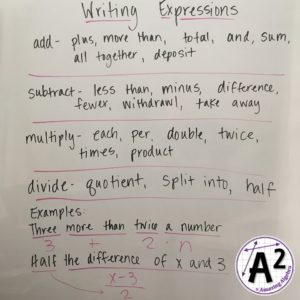Today we do a quick warm-up, review homework, continue the graphic organizer or foldable, practice problems in class, and then they do a homework assignment to reinforce the skills.

The practice problems can be used in a number of ways. You could copy them for students and work through them in order. You could pick problems to do on the board as you see how the students are doing. You could have the students put the problems on small dry erase boards or have them compare answers with an assigned partner next to them.

Writing expressions is the first step to doing word problems. They need to be able to write mathematical expressions from verbal ones. I usually start this lesson out with having the class list all the different ways to say add, subtract, multiply, and divide in math terms. I give hints for some of the more difficult ones and add a few of my own. Here are some examples that students have come up with in the past:I also show them how most of the time they can just writing the algebraic expression as they read the verbal expression. Sometimes I will just write it above so they can see it. The only special one to talk about is less than. I ask them what is 2 less than 5? Someone will say 3 and we write 5 – 2 = 3 on the board and talk about it. Less than is backwards from what we read, but makes logical sense if we use numbers.

One thing to point out is that two answers that look different can sometimes both be right.  For example:  2x - 3 is the same as -3 + 2x.  Equivalent expressions would be correct.

I provided a homework for this topic, but I usually just assign the study guide so we can review it the day before the test. That way the students can check answers and ask questions about their mistakes. I always stress that the study guides are very similar to the test and should be thought of as a practice test.